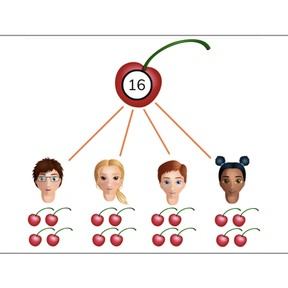# Adding equal amounts to 20

No account needed.8,000 schools use Gynzy92,000 teachers use Gynzy1,600,000 students use Gynzy

## General

Students learn to add equal amounts to 20 by splitting totals into equal groups of objects. They know how many each person will get if everyone gets the same amount.

2.OA.C

## Introduction

Set a number of blocks on the table and have the same number of students come to the front. Ask the rest of the class if there are equal amounts of blocks and students. Would everyone get a block? Repeat this with pencils and ask other students to come to the front.

## Instruction

On the interactive whiteboard, show equal amounts. There are equal amounts of football shoes and footballs. Ask two students to come to the front and give them a few erasers. Say that you can equally split the erasers between the two students. Give both students the erasers and ask them to count out loud how many they have. Say that they have the same amount or equal amounts. Then show a division of strawberries between two children. Show that there are multiple divisions of the strawberries and that both children still have the same amount. Then show how the bananas and cherries are equally split between the four children. Make sure to mention the addition problem with each of them, namely that the total is the combination of the equal amounts that each child gets.

Explain to students how to write this addition problem. The total number is written first, and then the addends are written.
Practice equally splitting numbers into groups with marbles and candies by dragging them to the child. Then write the addition problem that they represent. Next, there are images of children and objects. The students must fill in the missing numbers so that the objects are split equally between the children. Have students write their answers on a sheet of paper and hold them up to check their answers.

Check that students understand adding equal amounts to 20 by asking the following questions:
- What does it mean if you are going to split a number or total equally?
- How do you share objects in an equal way?

## Quiz

Students first practice equally splitting an amount between two children. Next, they are asked to equally split between three and four children.

## Closing

Discuss with students that it is important to learn how to equally split amounts so that everyone gets the same amount. Show a statement on the board- There is a situation with a number of students and a number of objects. Two children have an "answer". Students in class must decide which child is correct. Ask students to explain how they determined their answer.

## Teaching tips

Students who have difficulty with splitting totals into equal amounts can make use of MAB blocks or other manipulatives. Have them set out the blocks one by one, giving one to each child every time. Then count how many each child has.

## Instruction materials

Blocks, pencils, and erasers
Optional- other manipulatives

### The online teaching platform for interactive whiteboards and displays in schools

• Save time building lessons

• Manage the classroom more efficiently

• Increase student engagement